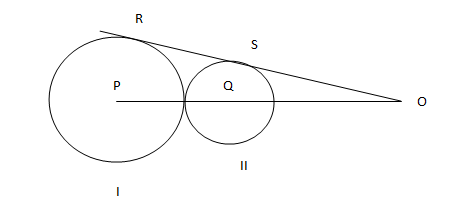### CAT 2004 Question Paper Question 2

Instructions

Directions for the following three questions: Answer the questions on the basis of the information given belowIn the adjoining figure I and II are circles with centres P and Q respectively, The two circles touch each other and have common tangent that touches them at points R and S respectively. This common tangent meets the line joining P and Q at O. The diameters of I and II are in the ratio 4: 3. It is also known that the length of PO is 28 cm.

Question 2

# What is the radius of the circle II?[CAT 2004]

Solution

Let the radius of circles I and II be 4R and 3R respectively.

Triangles PRO and QSO are similar.

PR/QS = 4/3.

Therefore, PO/QO = PR/QS

=> PO/QO = 4/3

=> 28/(28-7R) = 4/3

=> 4-R = 3

=> R = 1

Radius of smaller circle = 3R = 3